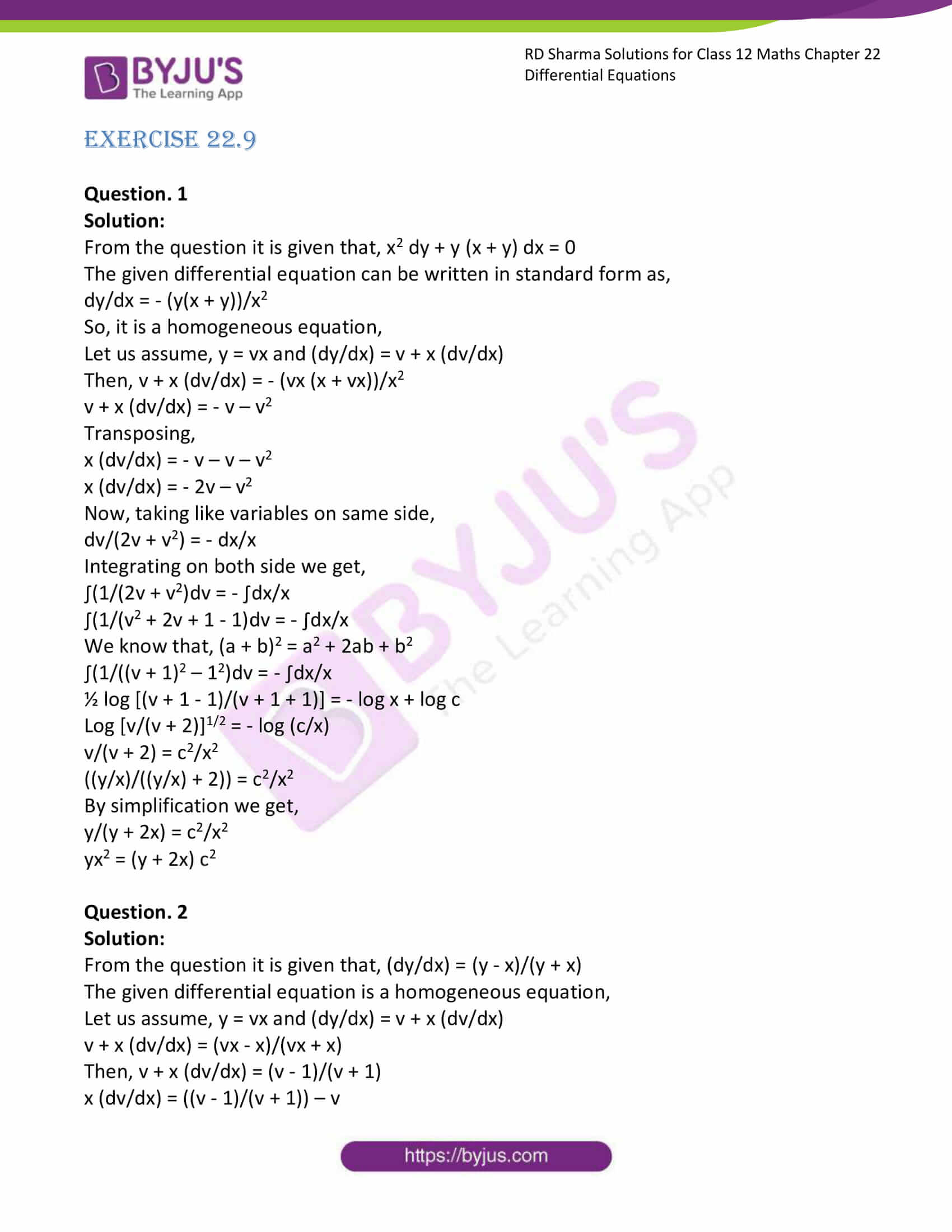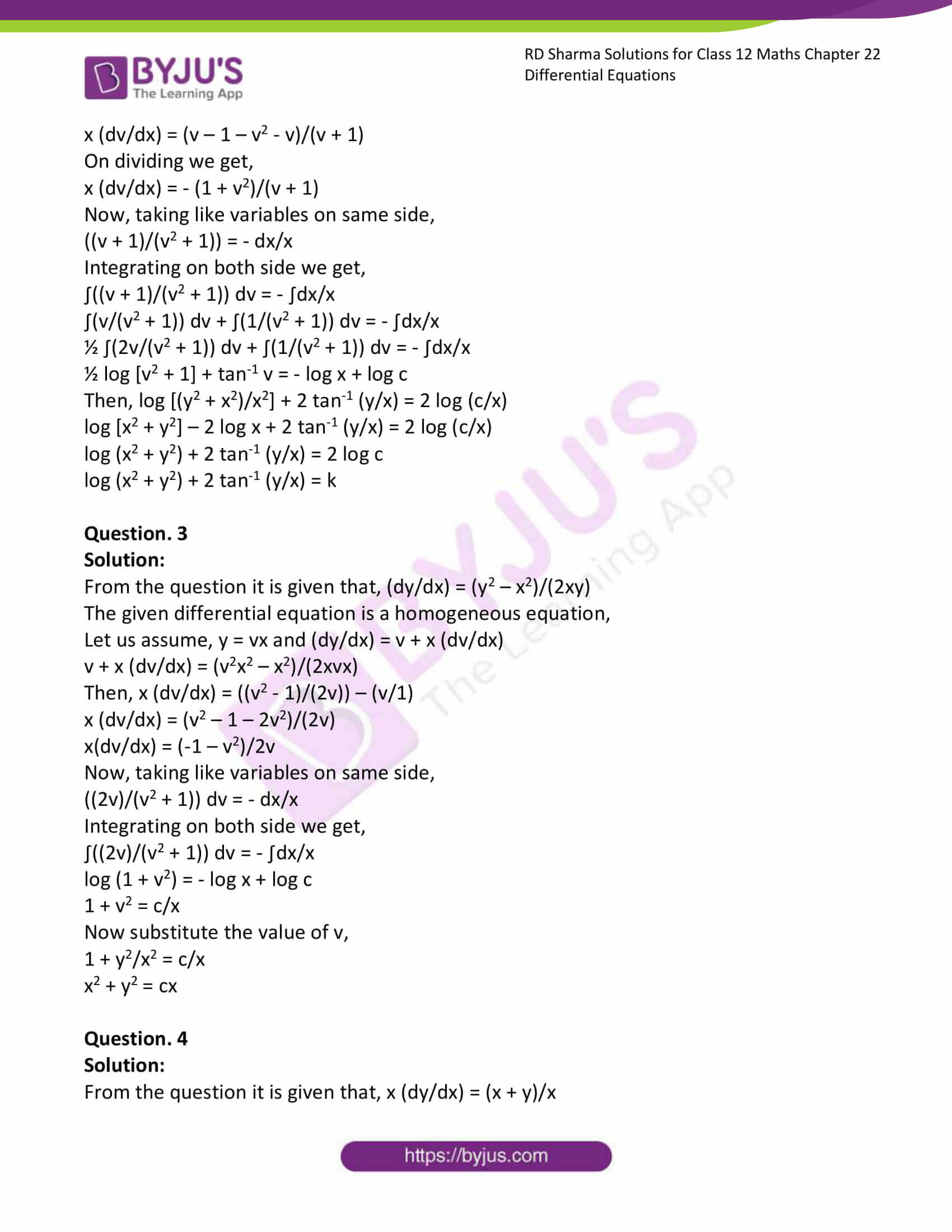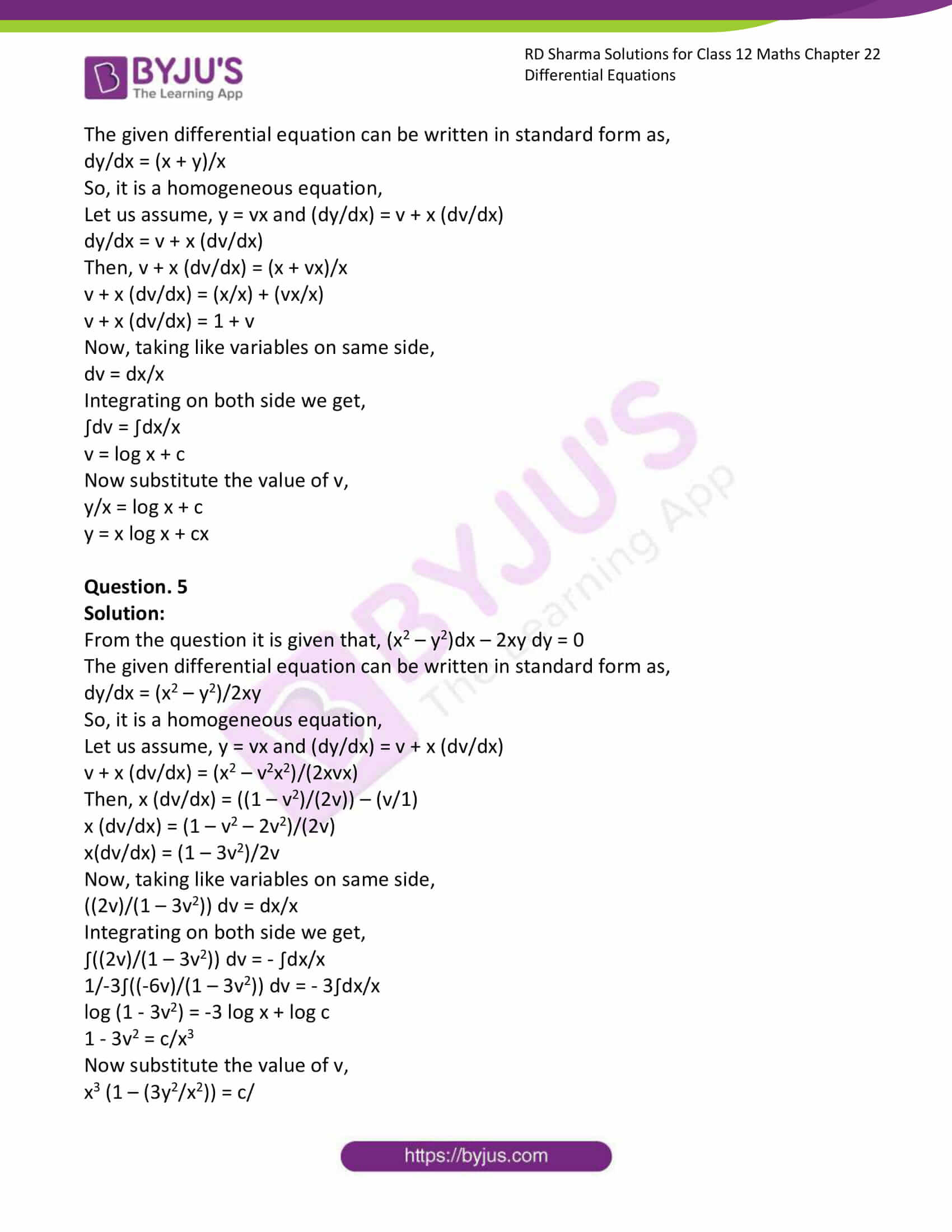# RD Sharma Solutions Class 12 Differential Equations Exercise 22.9

RD Sharma Solutions for Class 12 Maths Exercise 22.9 Chapter 22 Differential Equations are available here. While solving the exercise questions from the RD Sharma book, students often face difficulty and eventually pile up their doubts. Our expert tutors formulate this RD Sharma Solutions for Class 12 Maths Chapter 22 to assist you with your exam preparation to attain good marks in Maths.

## Download PDF of RD Sharma Solutions for Class 12 Maths Chapter 22 Exercise 9### Access RD Sharma Solutions for Class 12 Maths Chapter 22 Exercise 9

EXERCISE 22.9

Question. 1

Solution:

From the question it is given that, x2 dy + y (x + y) dx = 0

The given differential equation can be written in standard form as,

dy/dx = – (y(x + y))/x2

So, it is a homogeneous equation,

Let us assume, y = vx and (dy/dx) = v + x (dv/dx)

Then, v + x (dv/dx) = – (vx (x + vx))/x2

v + x (dv/dx) = – v – v2

Transposing,

x (dv/dx) = – v – v – v2

x (dv/dx) = – 2v – v2

Now, taking like variables on same side,

dv/(2v + v2) = – dx/x

Integrating on both side we get,

∫(1/(2v + v2)dv = – ∫dx/x

∫(1/(v2 + 2v + 1 – 1)dv = – ∫dx/x

We know that, (a + b)2 = a2 + 2ab + b2

∫(1/((v + 1)2 – 12)dv = – ∫dx/x

½ log [(v + 1 – 1)/(v + 1 + 1)] = – log x + log c

Log [v/(v + 2)]1/2 = – log (c/x)

v/(v + 2) = c2/x2

((y/x)/((y/x) + 2)) = c2/x2

By simplification we get,

y/(y + 2x) = c2/x2

yx2 = (y + 2x) c2

Question. 2

Solution:

From the question it is given that, (dy/dx) = (y – x)/(y + x)

The given differential equation is a homogeneous equation,

Let us assume, y = vx and (dy/dx) = v + x (dv/dx)

v + x (dv/dx) = (vx – x)/(vx + x)

Then, v + x (dv/dx) = (v – 1)/(v + 1)

x (dv/dx) = ((v – 1)/(v + 1)) – v

x (dv/dx) = (v – 1 – v2 – v)/(v + 1)

On dividing we get,

x (dv/dx) = – (1 + v2)/(v + 1)

Now, taking like variables on same side,

((v + 1)/(v2 + 1)) = – dx/x

Integrating on both side we get,

∫((v + 1)/(v2 + 1)) dv = – ∫dx/x

∫(v/(v2 + 1)) dv + ∫(1/(v2 + 1)) dv = – ∫dx/x

½ ∫(2v/(v2 + 1)) dv + ∫(1/(v2 + 1)) dv = – ∫dx/x

½ log [v2 + 1] + tan-1 v = – log x + log c

Then, log [(y2 + x2)/x2] + 2 tan-1 (y/x) = 2 log (c/x)

log [x2 + y2] – 2 log x + 2 tan-1 (y/x) = 2 log (c/x)

log (x2 + y2) + 2 tan-1 (y/x) = 2 log c

log (x2 + y2) + 2 tan-1 (y/x) = k

Question. 3

Solution:

From the question it is given that, (dy/dx) = (y2 – x2)/(2xy)

The given differential equation is a homogeneous equation,

Let us assume, y = vx and (dy/dx) = v + x (dv/dx)

v + x (dv/dx) = (v2x2 – x2)/(2xvx)

Then, x (dv/dx) = ((v2 – 1)/(2v)) – (v/1)

x (dv/dx) = (v2 – 1 – 2v2)/(2v)

x(dv/dx) = (-1 – v2)/2v

Now, taking like variables on same side,

((2v)/(v2 + 1)) dv = – dx/x

Integrating on both side we get,

∫((2v)/(v2 + 1)) dv = – ∫dx/x

log (1 + v2) = – log x + log c

1 + v2 = c/x

Now substitute the value of v,

1 + y2/x2 = c/x

x2 + y2 = cx

Question. 4

Solution:

From the question it is given that, x (dy/dx) = (x + y)/x

The given differential equation can be written in standard form as,

dy/dx = (x + y)/x

So, it is a homogeneous equation,

Let us assume, y = vx and (dy/dx) = v + x (dv/dx)

dy/dx = v + x (dv/dx)

Then, v + x (dv/dx) = (x + vx)/x

v + x (dv/dx) = (x/x) + (vx/x)

v + x (dv/dx) = 1 + v

Now, taking like variables on same side,

dv = dx/x

Integrating on both side we get,

∫dv = ∫dx/x

v = log x + c

Now substitute the value of v,

y/x = log x + c

y = x log x + cx

Question. 5

Solution:

From the question it is given that, (x2 – y2)dx – 2xy dy = 0

The given differential equation can be written in standard form as,

dy/dx = (x2 – y2)/2xy

So, it is a homogeneous equation,

Let us assume, y = vx and (dy/dx) = v + x (dv/dx)

v + x (dv/dx) = (x2 – v2x2)/(2xvx)

Then, x (dv/dx) = ((1 – v2)/(2v)) – (v/1)

x (dv/dx) = (1 – v2 – 2v2)/(2v)

x(dv/dx) = (1 – 3v2)/2v

Now, taking like variables on same side,

((2v)/(1 – 3v2)) dv = dx/x

Integrating on both side we get,

∫((2v)/(1 – 3v2)) dv = – ∫dx/x

1/-3∫((-6v)/(1 – 3v2)) dv = – 3∫dx/x

log (1 – 3v2) = -3 log x + log c

1 – 3v2 = c/x3

Now substitute the value of v,

x3 (1 – (3y2/x2)) = c/

x3 (x2 – 3y2)/x2 = c

x(x2 – 3y2) = c